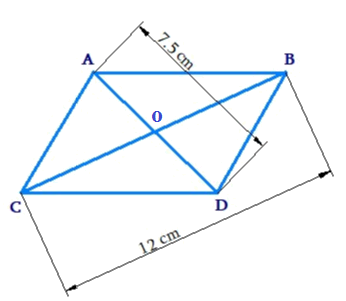In the verge of coronavirus pandemic, we are providing FREE access to our entire Online Curriculum to ensure Learning Doesn't STOP!

# Ex.11.2 Q5 Mensuration Solution - NCERT Maths Class 8

Go back to  'Ex.11.2'

## Question

The diagonals of a rhombus are $$7.5\,\rm{ cm}$$ and $$12\,\rm{ cm.}$$ Find its area.Video Solution
Mensuration
Ex 11.2 | Question 5

## Text Solution

What is Known?

Diagonals of the rhombus are given.

What is unknown?

Area of rhombus.

Reasoning:

By using the method of splitting into triangles (triangulation method) we can find area of the rhombus visually diagonals $$AD$$ and $$BC$$ are perpendicular bisectors of each other hence area of rhombus $$ABCD$$ will be the sum of area of triangle $$ACB$$ and area of the triangle $$DBC.$$

Steps:

Area of Rhombus $$ABCD$$

\begin{align} &= {\text{Area of }}\Delta \,ABC + {\text{Area of}}\,\Delta \,DCB\\ &= \frac{1}{2} \times (CB \times AO) + \frac{1}{2} \times (CB \times OD)\\&= \frac{}{2}CB \times (AO + OD)\\ &= \frac{1}{2} \times CB \times AD\\& = \frac{1}{2} \times 12\,{\rm{m}} \times 7.5\,{\rm{m}}\\ &= 45.0\,{{\rm{m}}^2}\end{align}

Thus, area of the rhombus is $$45.0\,{{\rm{m}}^2}$$

Learn from the best math teachers and top your exams

• Live one on one classroom and doubt clearing
• Practice worksheets in and after class for conceptual clarity
• Personalized curriculum to keep up with school